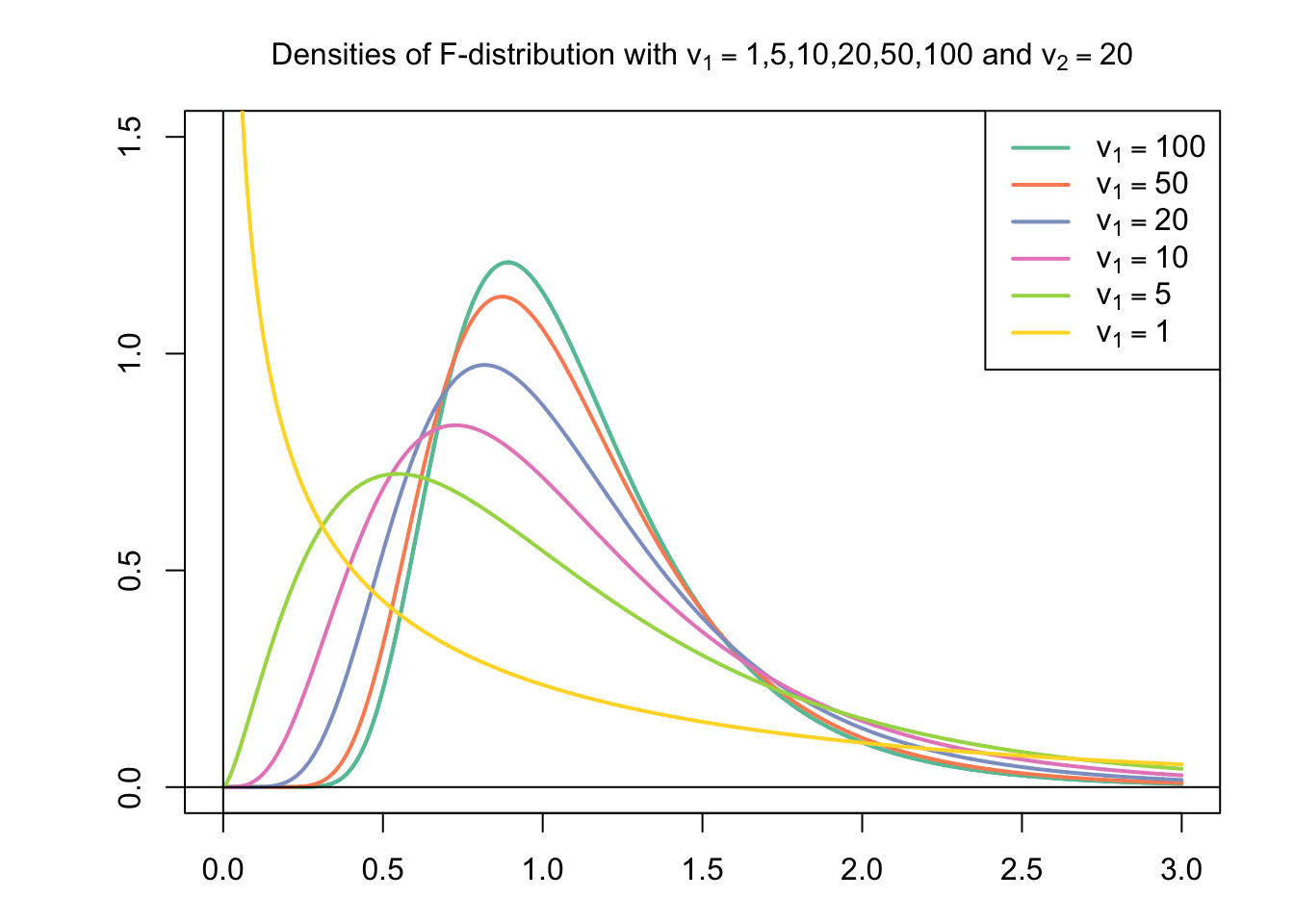The Snedecor’s $$F$$-distribution or the Fisher-Snedecor distribution (after Sir Ronald A. Fisher and George W. Snedecor) or short the $$F$$-distribution is a continuous probability distribution with range $$[0, +\infty)$$, depending on two parameters denoted $$v_1, v_2$$ (Lovric 2011). In statistical applications, $$v_1, v_2$$ are positive integers.

Let $$Y_1$$ and $$Y_2$$ be two independent random variables distributed as chi-square, with $$v_1$$ and $$v_2$$ degrees of freedom, respectively. Then the distribution of the ratio ($$Z$$)

$Z = \frac{Y_1/v_1}{Y_2/v_2}$

is called the $$F$$-distribution with $$v_1$$ and $$v_2$$ degrees of freedom. The $$F$$-distribution is often referred to as the distribution of the variance ratio (Lovric 2011).

A $$F$$-distribution has two numbers of degrees of freedom, $$v_1$$ and $$v_2$$, determining its shape. The first number of degrees of freedom, $$v_1$$, is called the degrees of freedom of the numerator and the second, $$v_2$$, the degrees of freedom of the denominator.